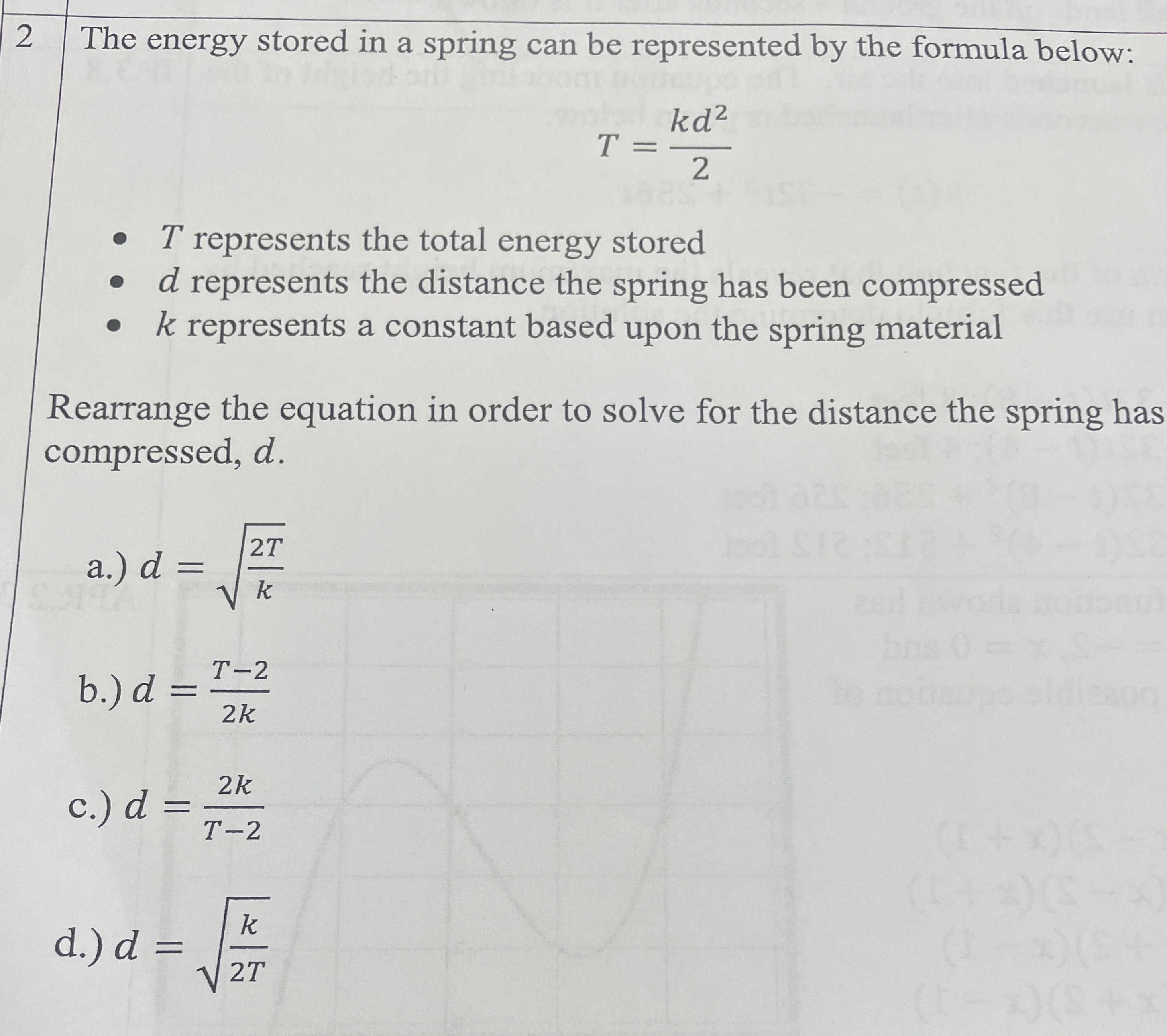### ¿Todavía tienes preguntas de matemáticas?

Pregunte a nuestros tutores expertos
Algebra
Pregunta$$2$$ The energy stored in a spring can be represented by the formula below: $$T = \frac { k d ^ { 2 } } { 2 }$$ .

$$T$$ represents the total energy stored .

$$d$$ represents the distance the spring has been compressed .

$$k$$ represents a constant based upon the spring material .

Rearrange the equation in order to solve for the distance the spring has compressed, $$d$$ .

a.) $$d = \sqrt { \frac { 2 T } { k } }$$

b.) $$d = \frac { T - 2 } { 2 k }$$

c.) $$d = \frac { 2 k } { T - 2 }$$

d.) $$d = \sqrt { \frac { k } { 2 T } }$$